A full review of each paper will be released after the seminar is finished. Meanwhile, here are questions that have been asked.

## Deep Reinforcement Learning with Double Q-learning

Double DQN

#### Q1. Why must states -6 and 6 always be included to avoid extrapolation?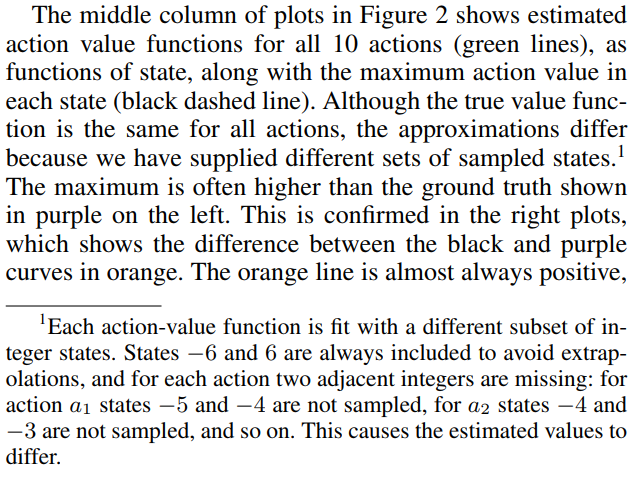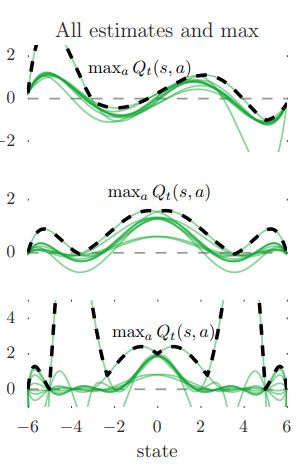Suppose that -6 is not sampled. Then, its value must be estimated from or some other close values. However, since values less than -6 cannot be sampled, it must be extrapolated only from one side: values more than -6. We want to test the amount of overestimation fairly, so we want all values to be intrapolated. If -6 and 6 is always included, any other value without samples can be intrapolated from some samples from below and some samples from above.

## Prioritized Experience Replay

PER

#### Q1. Why do the authors mention the power-law distribution while explaining the rank-based variant?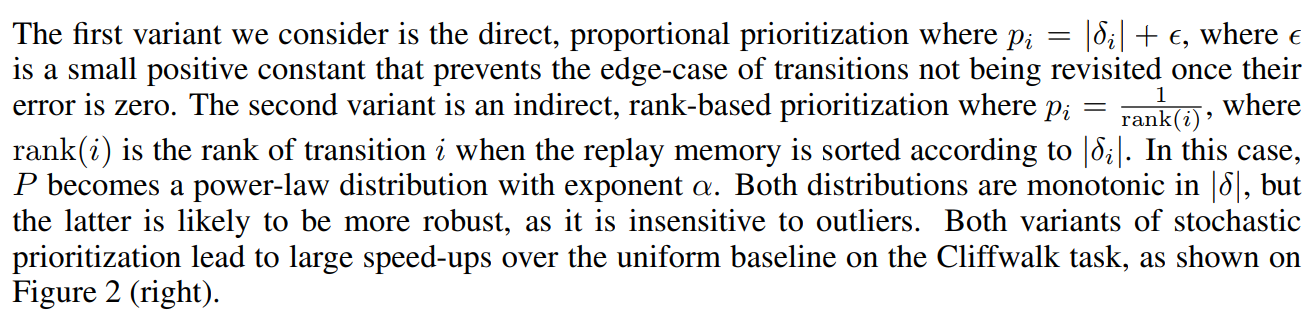The power-law distribution is monotonic, which might not be obvious with the equation $p_i = \frac{1}{\text{rank}(i)}$.

#### Q2. How does the Sum-Tree implementation work for proportional-based variant?To choose with priority, we need to first sum all the priority weights $W = \sum_i w_i$. Then, we select a random number $r$ from $[0, W]$. Finally, if $r \in [0, w_1]$, choice with weight $w_1$ is chosen. If $r \in [w_1, w_1 + w_2]$, choice with weight $w_2$ is chosen. In other words, if $r \in [\sum_{i=1}^k w_i, \sum_{i=1}^{k+1} w_i]$, choice with weight $w_{k+1}$ is chosen. The simplist possible data structure is an array $[w_1\;w_2\;w_3\;\ldots\;w_n]$. Then, to choose an element, we need to iteratively check if $r < \sum w_i$ for all $i$. This is inefficient, so we instead use the sum-tree implementation.

The sum-tree can be thought of as performing a binary search on the discrete CDF $\sum w_i$. In a tree structure, each parent node contains the sum of all its children. Then, to sample, we just need $O(\log n)$ inequality checks. This implementation does suffer from worse update complexity of $O(\log n)$, since the value of all parents and grandparents of the updated weight must also be updated. However, as a whole, it is faster than the naive array implementation.

## Dueling Network Architectures for Deep Reinforcement Learning

Dueling DQN

#### Q1. What is gradient clipping?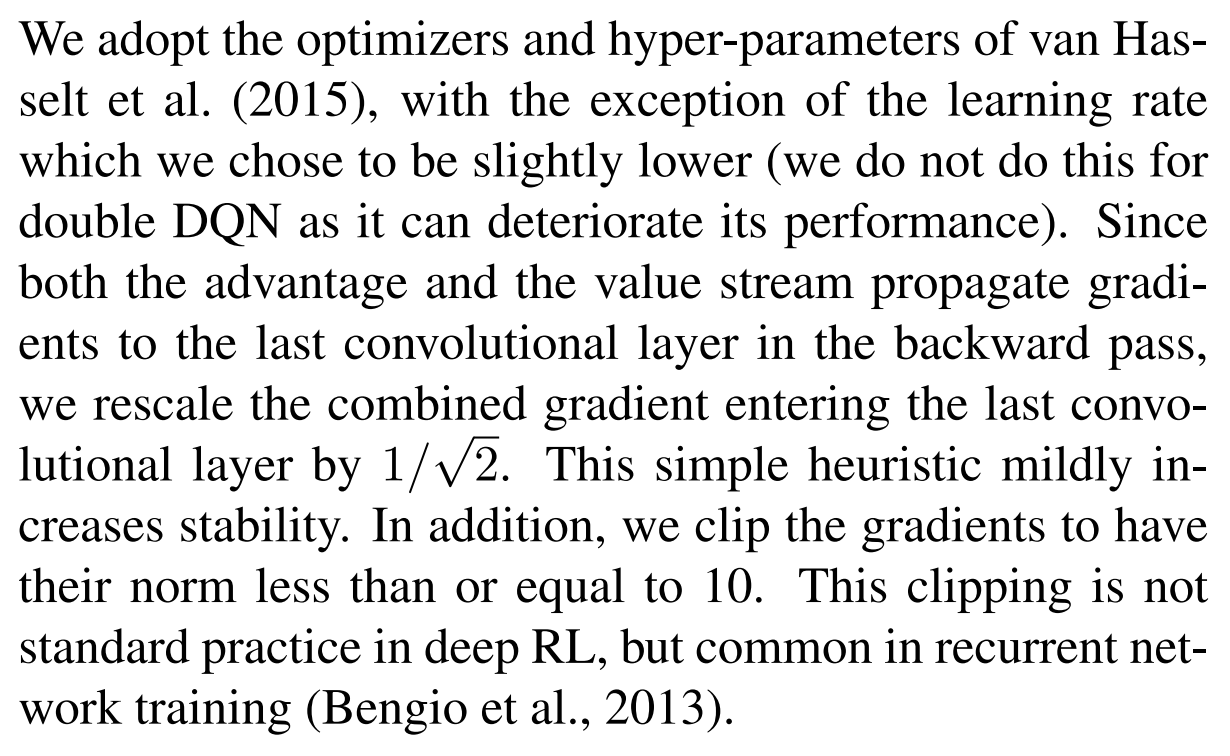Gradient clipping is a technique used most frequently for training deep neural networks or recurrent neural networks (RNNs) to prevent exploding gradients. When the error gradient is backpropagated long distances, the gradient can either “vanish” or “explode”. A vanishing gradient results in neural network weights not changing, while an exploding gradient results in instability or divergence. To mitigate such instability, it is a common technique to artificially limit the size of gradients. This is called “gradient clipping.”

#### Q2. Why is the naive aggregation module $Q = V + A$ unidentifiable?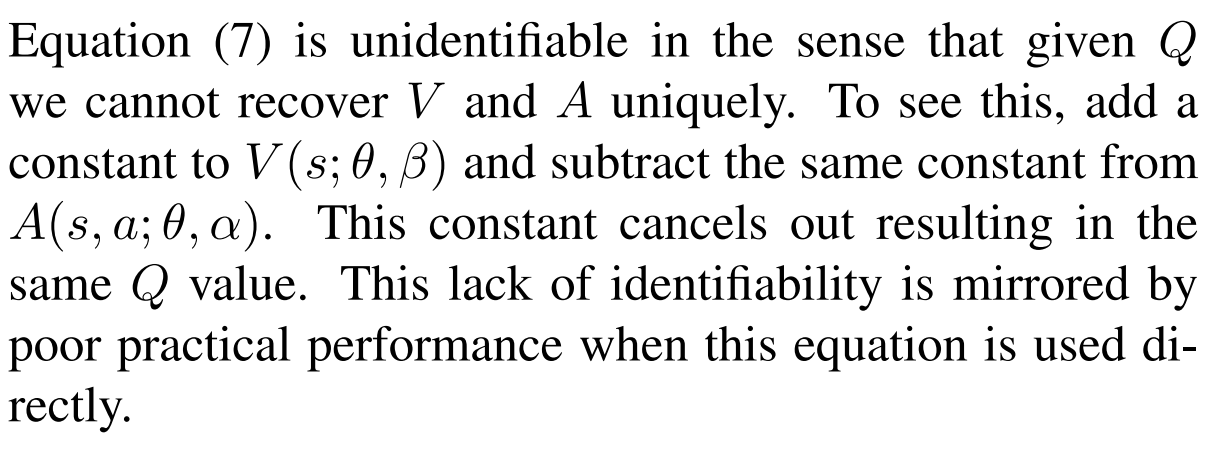Suppose that for some state $s$, $V_1(s; \theta, \beta) = x$ and $A_1(s, a_i; \theta, \alpha) = y_i$. Then, using the naive aggregating module $Q = V + A$, $Q_1(s, a_i; \theta, \alpha, \beta) = x + y_i$. Now, consider a different set of value estimate functions $V_2$ and $A_2$: $V_2(s; \theta, \beta) = x + \epsilon$ and $A_2(s, a_i; \theta, \alpha) = y_i - \epsilon$. Then $Q_2(s, a_i; \theta, \alpha, \beta) = x + y_i = Q_1(s, a_i; \theta, \alpha, \beta)$. Thus, different $V$ and $A$ can be aggregated to have same $Q$, so we cannot recover $V$ and $A$ from values of $Q$. Thus, this aggregation module is unidentifiable.